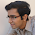## Tuesday, 23 October 2012

### 2n digit number

i) Consider a 4 digit number abcd. Will there exist a 'k' such that
abcd = k*ab*cd
If so, find the k and abcd?
ii) Now, consider a 6 digit number abcdef. Will there exist a 'k' such that
abcdef = k*abc*def
If so, find the k and abcdef?
iii) Using these 2 results, what can you conclude about a existence of a 'k' for a generic 2n digit number?

#### 1 comment:

1.i)
k=2, abcd= 1352
k=3, abcd= 1734

ii)
k=7, abcdef=143143
k=3, abcdef=167334

iii)
I will explain it for a 6 digit number. The logic for a 2n digit number is quite similar.

abcdef should be divisible by abc. By writing abcdef as 1000*abc + def, we see that def should be divisible by abc. Let def=m*abc, for some single digit number m.

When we divide abcdef by abc, the quotient is 10^3+m. We are left with def*k in the RHS.
Let M=10^3 +m. Therefore the equation we are left with is:

M = k*def
or M=k*m*abc.

Therefore, M needs to be divisible by m. Let D=M/m. Then we are left with

D =k*abc.

We cannot take abc = D because m*D=M, which is a 4 digit number. Therefore, abc can be any 3 digit proper divisor of D, and k=D/abc. def=m*abc. (We will have to try 9 possible values of m)

Example:

i)
M=1001, m=1, D=1001
D=13*11*7
D has 143 as its only 3 digit proper divisor.
Hence, abc=143, k=7 and def=143*1=143.
abcdef=143143, k=7.

ii)
M=1002, m=2, D=501.
D=167*3
The only 3 digit proper divisor of 501 is 167.
abc=167, k=3 and def=167*2=334.
abcdef=167334.